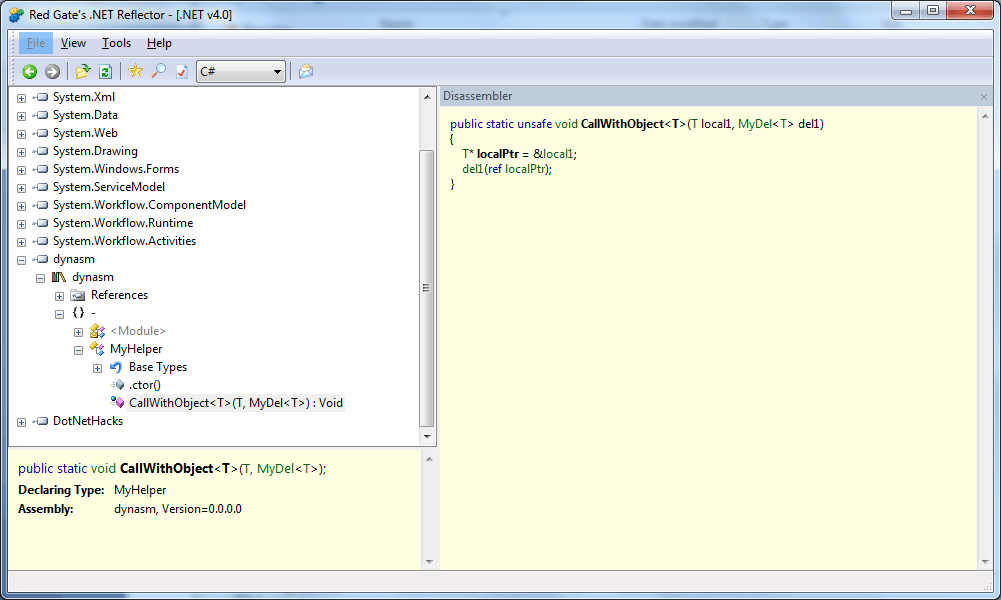Disclaimer: this post is all about fun. It is not about:

•   turning a bug into a feature
•   some new isolation/mocking practice
•   wow, look what I will do with pointers in my next project

It is all about fun :) It shows some of CLR internals as well.

As far as I know since .NET 2.0 there is a bug in Microsoft C# compiler that makes it possible to compile the following source code.

`class Class1`
`{`
`    ``unsafe public static void Method1(ref object* obj) {}`
`}`

Trying to compile it with .NET 1.1 Microsoft C# compiler produces an error:

`error CS1005: Indirection to managed type is not valid`

In my job I have to use both C++ and C# and sometimes switching the context is not easy. C# is lovely language and it is really fun to work with. So I thought wouldn't it be fun to use C++ style pointers as well. That's how I came up with the idea for this posting. Well let me show you a demo program first and then I will explain it in details.

`using` `System;`
`using` `System.Reflection;`
`using` `System.Reflection.Emit;`
`using` `System.Runtime.InteropServices;`

`namespace` `DotNetHacks`
`{`
`    ``unsafe` `public` `delegate` `void` `MyDel<T>(``ref` `T* obj);`

`    ``public` `class` `MyClass`
`    ``{`
`        ``public` `void` `SayHi(``string` `text)`
`        ``{`
`            ``Console.WriteLine(``"Hi {0}!"``, text);`
`        ``}`

`        ``public` `void` `SayHello(``string` `text)`
`        ``{`
`            ``Console.WriteLine(``"Hello {0}!"``, text);`
`        ``}`
`    ``}`

`    ``unsafe` `class` `Program`
`    ``{`
`        ``public` `static` `void` `CreateDynAsm()`
`        ``{`
`            ``var app = AppDomain.CurrentDomain;`

`            ``var filename = ``"dynasm"``;`

`            ``var asmName = ``new` `AssemblyName(filename);`

`            ``var asmBld = app.DefineDynamicAssembly(asmName, AssemblyBuilderAccess.RunAndSave);`

`            ``var modBld = asmBld.DefineDynamicModule(filename, filename + ``".dll"``);`

`            ``var typeBld = modBld.DefineType(``"MyHelper"``, TypeAttributes.Class | TypeAttributes.Public | TypeAttributes.Sealed | TypeAttributes.AnsiClass | TypeAttributes.AutoClass);`

`            ``var methBld = typeBld.DefineMethod(``"CallWithObject"``, MethodAttributes.Public | MethodAttributes.HideBySig | MethodAttributes.Static);`

`            ``var genBld = methBld.DefineGenericParameters(``"T"``);`

`            ``var myDelGenType = ``typeof``(MyDel<>);`

`            ``methBld.SetParameters(genBld, myDelGenType.MakeGenericType(genBld));`
`            ``methBld.SetReturnType(``typeof``(``void``));`

`            ``var il = methBld.GetILGenerator();`

`            ``var locBld = il.DeclareLocal(genBld.MakePointerType());`

`            ``il.Emit(OpCodes.Ldarga_S, 0);`
`            ``il.Emit(OpCodes.Stloc_0);`
`            ``il.Emit(OpCodes.Ldarg_1);`
`            ``il.Emit(OpCodes.Ldloca_S, 0);`
`            ``il.Emit(OpCodes.Callvirt, TypeBuilder.GetMethod(myDelGenType.MakeGenericType(genBld), myDelGenType.GetMethod(``"Invoke"``)));`
`            ``il.Emit(OpCodes.Ret);`

`            ``var type = typeBld.CreateType();`

`            ``asmBld.Save(asmName.Name + ``".dll"``);`
`        ``}`

`        ``public` `static` `void` `SayHello(``ref` `MyClass* obj)`
`        ``{`
`            ``obj->SayHello(``"John"``);`

`            ``// print some info that we can verify with SOS.DLL`

`            ``IntPtr objRef = Marshal.ReadIntPtr(``new` `IntPtr(obj));`

`            ``Console.WriteLine(``"objRef = "` `+ ((IntPtr.Size == 4)`
`                                  ``? objRef.ToInt32().ToString(``"X"``)`
`                                  ``: objRef.ToInt64().ToString(``"X"``)));`
`            `
`            ``bool` `success = ``true``;`

`            ``if` `(IntPtr.Size == 4)`
`            ``{`
`                ``int` `typeHandle = Marshal.ReadInt32(objRef);`
`                ``Console.WriteLine(``"typeHandle = "` `+ typeHandle.ToString(``"X"``));`

`                ``success = typeHandle == ``typeof``(MyClass).TypeHandle.Value.ToInt32();`
`            ``}`
`            ``else`
`            ``{`
`                ``long` `typeHandle = Marshal.ReadInt64(objRef);`
`                ``Console.WriteLine(``"typeHandle = "` `+ typeHandle.ToString(``"X"``));`

`                ``success = typeHandle == ``typeof``(MyClass).TypeHandle.Value.ToInt64();`
`            ``}`

`            ``if` `(!success)`
`            ``{`
`                ``throw` `new` `Exception(``"something wrong"``);`
`            ``}`
`        ``}`

`        ``public` `static` `void` `SayHelloAgain(``ref` `MyClass* obj)`
`        ``{`
`            ``if` `(IntPtr.Size == 4)`
`            ``{`
`                ``/* 1 */` `int``* funcPtr = (``int``*)``typeof``(MyClass).GetMethod(``"SayHi"``).MethodHandle.Value.ToPointer();`
`                ``/* 2 */` `funcPtr += 2; ``// 8 bytes`
`                ``/* 3 */` `*funcPtr = ``typeof``(MyClass).GetMethod(``"SayHello"``).MethodHandle.GetFunctionPointer().ToInt32();`
`            ``}`
`            ``else`
`            ``{`
`                ``/* 1 */` `long``* funcPtr = (``long``*)``typeof``(MyClass).GetMethod(``"SayHi"``).MethodHandle.Value.ToPointer();`
`                ``/* 2 */` `funcPtr += 1; ``// 8 bytes`
`                ``/* 3 */` `*funcPtr = ``typeof``(MyClass).GetMethod(``"SayHello"``).MethodHandle.GetFunctionPointer().ToInt64();`
`            ``}`

`            ``obj->SayHi(``"Dave"``);`
`        ``}`

`        ``public` `static` `void` `NextDouble(``ref` `Random* obj)`
`        ``{`
`            ``Console.WriteLine(obj->NextDouble());`
`        ``}`

`        ``static` `void` `Main(``string``[] args)`
`        ``{`
`            ``CreateDynAsm(); ``return``;`

`            ``//MyHelper.CallWithObject(new Random(), NextDouble);`
`            ``//MyHelper.CallWithObject(new MyClass(), SayHello);`
`            ``//MyHelper.CallWithObject(new MyClass(), SayHelloAgain);`
`        ``}`
`    ``}`
`}`

I wondered how I can call a method with signature like Method1. I haven't found quick solution so I decided to emit a new assembly that I can reference later in the build process. First you have to run the program that has Main method like this one:

`CreateDynAsm(); ``return``;`

`//MyHelper.CallWithObject(new Random(), NextDouble);`
`//MyHelper.CallWithObject(new MyClass(), SayHello);`
`//MyHelper.CallWithObject(new MyClass(), SayHelloAgain);`

CreateDynAsm() will produce new assembly called dynasm.dll and then you have to reference that assembly from Visual Studio. Let’s examine with .NET Reflector the content of dynasm.dll assembly.As we can see there is not much. There is only method CallWithObject that gets the address of the first parameter and calls the delegate with it.

Once we have added dynasm.dll as a reference in Visual Studio we can modify our Main method to look like this one:

`//CreateDynAsm(); return;`

`MyHelper.CallWithObject(``new` `Random(), NextDouble);`
`MyHelper.CallWithObject(``new` `MyClass(), SayHello);`
`MyHelper.CallWithObject(``new` `MyClass(), SayHelloAgain);`

Let’s set a break point at the end of SayHello method and run the project in Debug mode. Now lets execute some commands in Immediate window (see the screenshot).

`.load sos.dll`
`!DumpObj 23BD048`

First I execute “.load sos.dll” and then execute “!DumpObj 23BD048” where the argument of DumpObj command is the number shown on the screen. This clearly shows that “obj” parameter points to a valid instance.

So far we saw how can use C++ style pointer syntax in C#. As you know I worked on JustMock product and more specifically on a component that rewrites MSIL on the fly. However this requires to have a running profiler. For simple scenarios I would like to show you another approach that is very similar to the approach used by Ziad Elmalki. We can substitute the actual function pointer for a given method. Lets looks at SayHelloAgain method. All we have to do is:

1)   Get pointer to MethodDesc structure for the target method (in our case SayHi)
2)   Find the offset where the actual function pointer is stored
3)   Overwrite the function pointer with the function pointer of the substitute (in our case SayHello)

Now, every time method SayHi is invoked method SayHello is be actually executed.